Study Materials: ncert solutions

Our ncert solutions for Exercise 6.1 Class 9 maths 6. Lines and Angles - ncert solutions - Toppers Study is the best material for English Medium students cbse board and other state boards students.

Solutions ⇒ Class 9th ⇒ Mathematics ⇒ 6. Lines and Angles

# Exercise 6.1 Class 9 maths 6. Lines and Angles - ncert solutions - Toppers Study

Topper Study classes prepares ncert solutions on practical base problems and comes out with the best result that helps the students and teachers as well as tutors and so many ecademic coaching classes that they need in practical life. Our ncert solutions for Exercise 6.1 Class 9 maths 6. Lines and Angles - ncert solutions - Toppers Study is the best material for English Medium students cbse board and other state boards students.

## Exercise 6.1 Class 9 maths 6. Lines and Angles - ncert solutions - Toppers Study

CBSE board students who preparing for class 9 ncert solutions maths and Mathematics solved exercise chapter 6. Lines and Angles available and this helps in upcoming exams 2023-2024.

### You can Find Mathematics solution Class 9 Chapter 6. Lines and Angles

• All Chapter review quick revision notes for chapter 6. Lines and Angles Class 9
• NCERT Solutions And Textual questions Answers Class 9 Mathematics
• Extra NCERT Book questions Answers Class 9 Mathematics
• Importatnt key points with additional Assignment and questions bank solved.

NCERT Solutions do not only help you to cover your syllabus but also will give to textual support in exams 2023-2024 to complete exercise 6.1 maths class 9 chapter 6 in english medium. So revise and practice these all cbse study materials like class 9 maths chapter 6.1 in english ncert book. Also ensure to repractice all syllabus within time or before board exams for ncert class 9 maths ex 6.1 in english.

See all solutions for class 9 maths chapter 6 exercise 6 in english medium solved questions with answers.

### Exercise 6.1 class 9 Mathematics Chapter 6. Lines and Angles

• Exercise 6.1 Class 9 Maths 6. Lines And Angles - Ncert Solutions - Toppers Study
• Class 9 Ncert Solutions
• Solution Chapter 6. Lines And Angles Class 9
• Solutions Class 9
• Chapter 6. Lines And Angles Exercise 6.1 Class 9

## Exercise 6.1 Class 9 maths 6. Lines and Angles - ncert solutions - Toppers Study

Exercise 6.1

Q1.  In Fig. 6.13, lines AB and CD intersect at O. If  AOC + ∠ BOE = 70° and ∠BOD = 40° find ∠BOE and reflex COE.

Solution: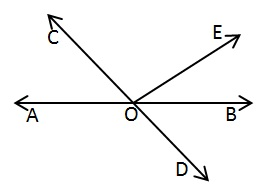∠BOD = 40°

AOC  = ∠BOD (Vertically opposite Angle)

AOC = 40°

AOC  + ∠ BOE = 70° (Given)

BOE = 70°

BOE = 70° - 40°

∠BOE = 30°

AOB is straight line

AOC +  COE +BOE = 180° (linear pair)

⇒ 70° + ∠COE = 180°

∠COE = 180° - 70°

∠COE = 110°

Reflex ∠COE = 360 - 110°

= 250°

Q2. In Fig. 6.14, lines XY and MN intersect at O. If POY = 90° and a : b = 2 : 3, find c.

Solution:POY=90° (given)

Let  a and b = 2x and 3x

XOY is a straight line

a + b + POY = 180°

2x + 3x + 90°= 180°

5x  = 180° ­­- 90°

5x = 90°

x = 90°/5

x = 18°

Now a = 2 x 18°

= 36°

b =3 x 18°

= 54°

MON is a straight line

b + c = 180°(linear pair)

54° + c = 180°

⇒∠c = 180°- 54°

=126°

Q3. In Fig. 6.15, ∠PQR = ∠PRQ, then prove that ∠PQS = ∠PRT

Solution :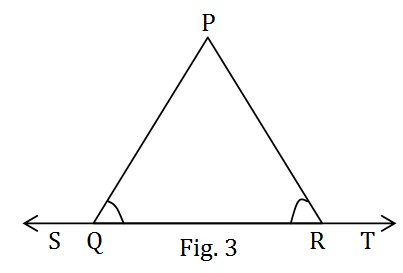Given : ∠PQR = ∠PRQ

To prove : ∠PQS = ∠PRT

Proof :

∠PQS + ∠PQR = 180°  .................. (1)  Linear pair

∠PRT + ∠PRQ = 180°  .................. (2)  Linear pair

From equation (1) and (2)

∠PQS + ∠PQR = ∠PRT + ∠PRQ

Or,  ∠PQS + ∠PQR = ∠PRT + ∠PQR    (∠PQR = ∠PRQ given)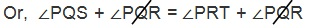Or, ∠PQS = ∠PRT Proved

Q4. In Fig. 6.16, if x + y = w + z, then prove that AOB is a line.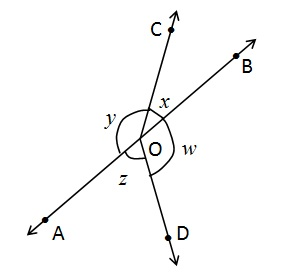Solution:

Given : x + y = w + z

To prove : AOB is a line.

Proof :

We know that;

x + y + w + z = 360

(Angle Sustained on centre)

x + y + x + y =  360 (x + y = w + z given)

2x + 2y = 360

2 (x + y) = 360

x + y = 180 (linear pair)

Therefore, AOB is a line

Hence, Proved

Q5. In Fig. 6.17, POQ is a line. Ray OR is perpendicular to line PQ. OS is another ray lying between rays OP and OR. Prove that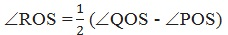Solution:

Given:

POQ is a straight line. OR PQ and OS is

another ray lying between rays OP and OR.

To prove:Proof: OR PQ  (given)

∴ ∠QOR = 90  …………… (1)

POQ is straight line

∴ ∠POR + QOR = 180 (linear pair)

POR + 90 = 180

POR = 180– 90

POR = 90…………… (2)

Now,  ROS + QOR = QOS

Or,       ROS = QOS – QOR  ……………. (3)

Again, ROS + POS = POR

Or,       ROS = POR – POS  ……………. (4)

ROS + ROS = QOS – QOR + POR – POS

2 ROS = QOS – 90+ 90POS

2 ROS = (QOS – POS)Hence Proved

Q6.  It is given that ∠ XYZ = 64° and XY is produced to point P. Draw a figure from the given information. If ray YQ bisects ∠ ZYP, find ∠ XYQ and reflex ∠ QYP.

Solution:Given: ∠ XYZ = 64°and XY is produced to point P. YQ bisects ∠ ZYP.

To Find: ∠XYQ and reflex ∠QYP.

YQ bisects ∠ZYP

∴ ∠ZYQ = ∠QYP   ................. (1)

∵ XY is produced to point P.

∴ PX is a straight line.

Now, ∠ XYZ + ∠ZYQ + ∠QYP = 180° (linear pair)

Or,     64° + ∠ZYQ + ∠QYP = 180°

⇒      ∠ZYQ + ∠QYP = 180° - 64°

⇒      ∠ZYQ + ∠ZYQ = 116°                 [Using equation (1) ]

⇒      2∠ZYQ = 116°

⇒      ∠ZYQ = 116°/2

⇒      ∠ZYQ = 58°

∠ZYQ = ∠QYP = 58°

∠XYQ = ∠XYZ + ∠ZYQ

= 64° + 58°

= 122°

∵ ∠QYP = 58°

∴ Reflex ∠QYP = 360° - 58°

= 302°

∠XYQ  = 122°, Reflex ∠QYP = 302°

##### Other Pages of this Chapter: 6. Lines and Angles

Important Study materials for classes 06, 07, 08,09,10, 11 and 12. Like CBSE Notes, Notes for Science, Notes for maths, Notes for Social Science, Notes for Accountancy, Notes for Economics, Notes for political Science, Noes for History, Notes For Bussiness Study, Physical Educations, Sample Papers, Test Papers, Mock Test Papers, Support Materials and Books.Mathematics Class - 11th

NCERT Maths book for CBSE Students.

books

## Study Materials List:

##### Solutions ⇒ Class 9th ⇒ Mathematics
1. Number Systems
2. Polynomials
3. Coordinate Geometry
4. Linear Equation In Two Variables
5. Introduction To Euclid’s Geometry
6. Lines and Angles
7. Triangles